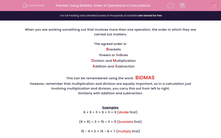# Using BIDMAS: Order of Operations in Calculations

In this worksheet, students must pay attention to the order of priority in carrying out mixed operations.This content is premium and exclusive to EdPlace subscribers.Key stage:  KS 2

Curriculum topic:   Maths and Numerical Reasoning

Curriculum subtopic:   Mixed Problems

Difficulty level:#### Worksheet Overview

When you are working something out that involves more than one operation, the order in which they are carried out matters.

The agreed order is:

Brackets
Powers or Indices
Division and Multiplication

This can be remembered using the word:  BIDMAS

However, remember that multiplication and division are equally important, so in a calculation just involving multiplication and division, you carry this out from left to right.

Examples

6 + 9 ÷ 3 = 6 + 3 = 9 (divide first)

(6 + 9) ÷ 3 = 15 ÷ 3 = 5 (brackets first)

15 - 4 × 2 = 15 - 8 = 7 (multiply first)

(15 - 4) × 2 = 11 × 2 = 22 (brackets first)

4 × 32 = 4 × 9 = 36 (power first)

(4 × 3)2 = 122 = 144 (brackets first)

14 - 4 + 5 = 15 (equally important, so left to right)

### What is EdPlace?

We're your National Curriculum aligned online education content provider helping each child succeed in English, maths and science from year 1 to GCSE. With an EdPlace account you’ll be able to track and measure progress, helping each child achieve their best. We build confidence and attainment by personalising each child’s learning at a level that suits them.

Get started